# What Is a Capacitive Voltage Divider?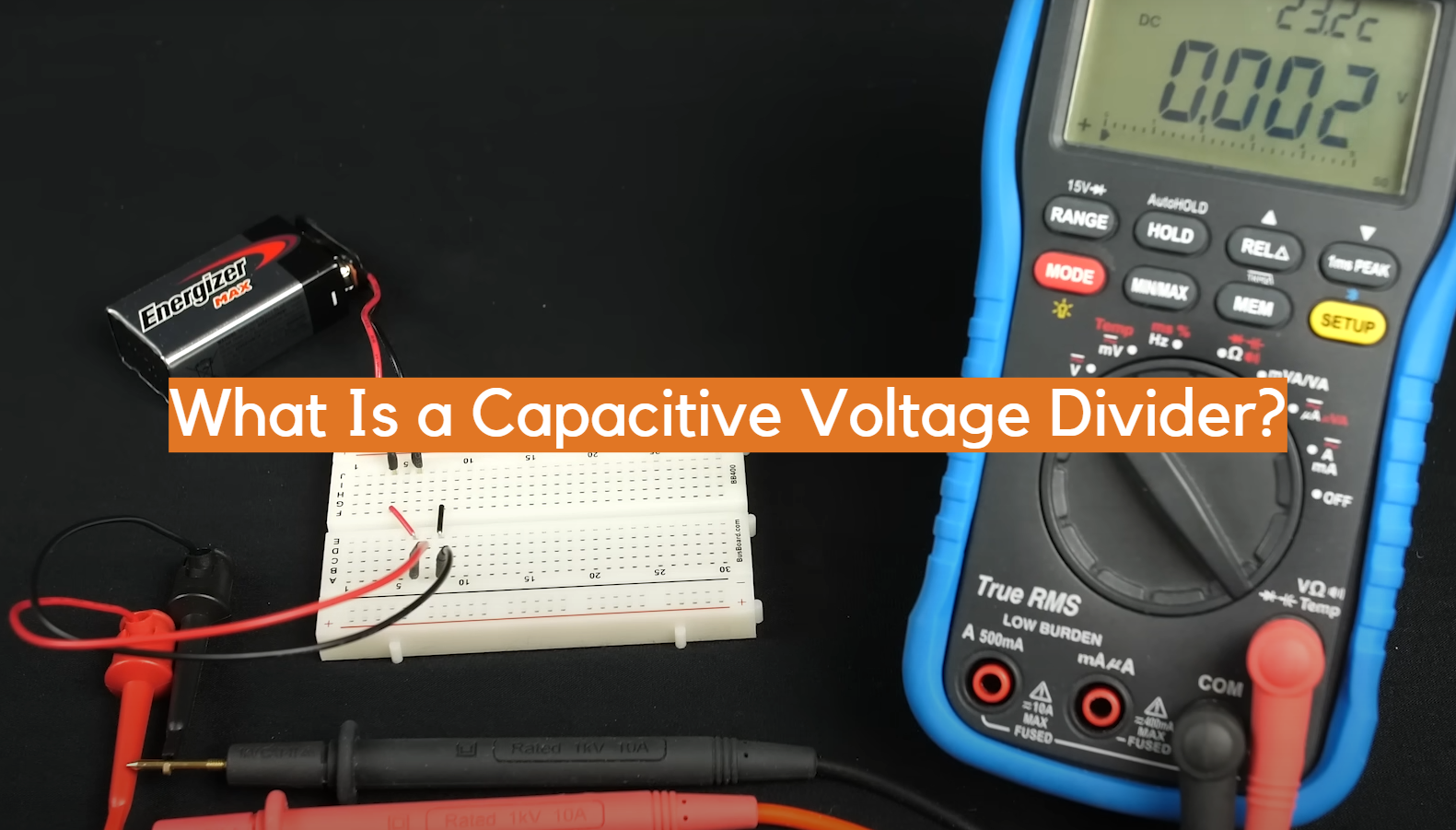Have you ever wondered how your electronic devices manage to divide voltages? It’s all thanks to capacitive voltage dividers! These nifty little circuits are used in a wide range of applications, from power supplies to sensor networks. In this blog post, we’ll take a closer look at how they work and some of the different ways they can be used. Stay tuned to learn more about this essential piece of electronics hardware!

## What is Capacitive Voltage Divider?

By connecting two or more capacitors in series, you can construct a Capacitive Voltage Divider that provides electric energy to your circuit. The voltage appearing across each capacitor is directly proportional to its capacitance, while the overall voltage is distributed between them based on their respective values. This type of divider can be used to create a single output voltage from multiple input sources, or to provide isolation between circuits with different voltages. Capacitive voltage dividers are common components in power supplies, audio systems, and other applications where accurate control of DC voltages is needed.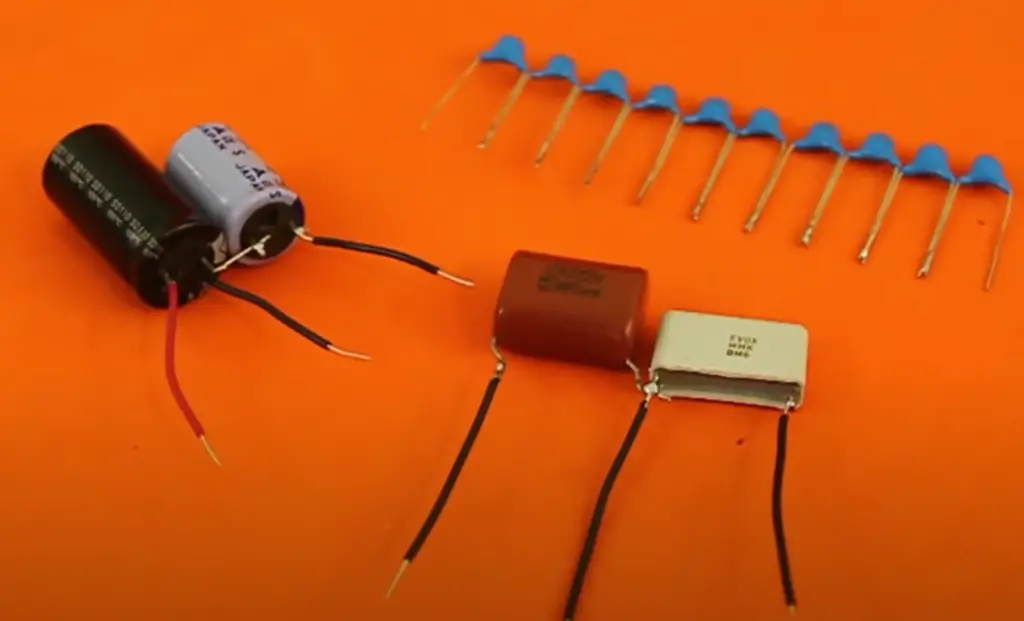## How to Work Capacitive Voltage Divider?

By placing two capacitors in a series along with a resistor, you can build an efficient and effective capacitive voltage divider. The first capacitor is connected to the input voltage, while the second is connected to ground. The ratio of voltage across the two capacitors can be adjusted by varying the resistance values in between them. As current flows through the resistors, it creates an electrical field that affects how much charge each capacitor holds and as a result, changes their relative voltages. This allows for precision tuning of voltage levels in circuits without physically adjusting any components. By properly selecting the right combination of capacitance and resistance values, engineers can create highly accurate voltage dividers for specific applications.

In essence, a capacitive voltage divider helps to reduce or increase the magnitude of a given voltage by distributing it between two capacitors and a resistor. This technique can be used in applications such as power supply regulation, dimming LED lighting systems, or noise cancellation. By adjusting the values of these components, different ratios can be achieved to create precision levels of voltage distribution. 

## Capacitive Voltage Divider Circuit

By substituting capacitors for resistors, a Capacitive Voltage Divider Circuit offers an innovative way to divide electrical voltages. This type of circuit is used in many electronic devices and appliances, such as power supplies, computers, TVs, and more. The basic function of a capacitive voltage divider is to take an input voltage and divide it into two output voltages that are proportional to each other. For example, if the input voltage is 10V then the output voltages can be 8V and 2V respectively.

The way this works is by using the capacitance of each capacitor in the series to create a division between input and output voltages. When working with a capacitive voltage divider circuit there are three major components involved: the input voltage source, the capacitors, and the output voltage. The input voltage is applied to one capacitor and then it is divided by each of the other two capacitors in the series. This creates two output voltages that are proportional to each other based on their individual capacitance values.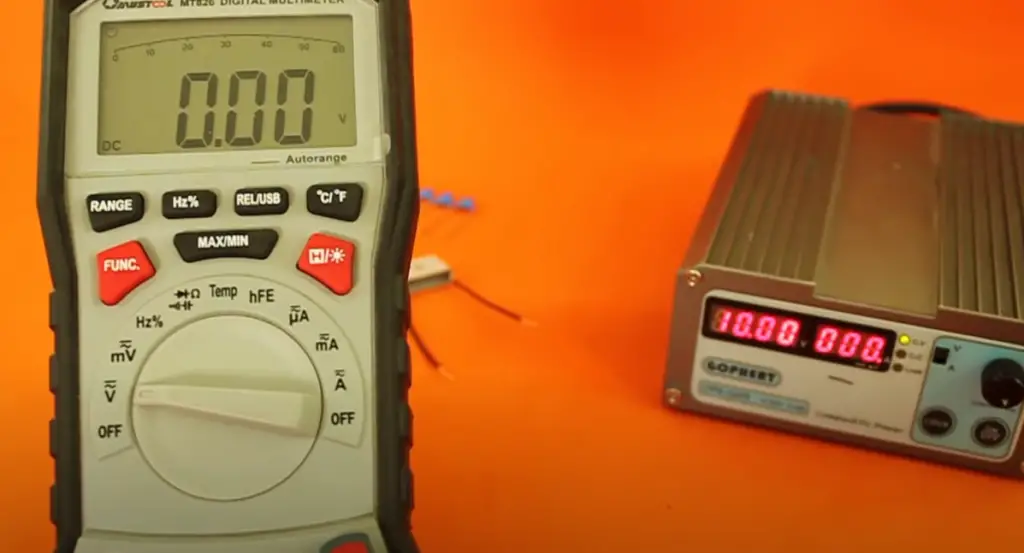## Example Problems

Example problems can be solved by using a capacitive voltage divider. In this example, we will use a resistor and capacitor combination to create a simple series circuit that is driven by an AC voltage source. The goal is to determine the output voltage across the capacitor under various conditions.

Let’s start with a basic situation – given two resistors (R1=50 ohms, and R2=100 ohms) in series with a capacitor (C = 1 microfarad) and connected to an AC voltage source (Vin = 10 volts). Using Kirchhoff’s Voltage Law, we can compute the total current in the circuit: It = Vin/(R1 + R2), which gives us 0.166 amps.

Next, we can use the current to calculate the voltage across each component in the circuit by applying Ohm’s Law, Vc = It x C, which yields 1.66 volts across the capacitor. This voltage is also known as the output voltage of the capacitive voltage divider. Therefore, using a capacitive voltage divider with two resistors and one capacitor connected to an AC source can yield an output of 1.66 volts.

In another example problem, let’s assume that R1 and R2 remain unchanged (50 ohms and 100 ohms) but now there are two capacitors connected in parallel (C1 = 0.5 microfarad, and C2 = 0.75 microfarad). Now, the total capacitance is the sum of both (C = 1.25 microfarads), and we can calculate the output voltage again using Kirchhoff’s Voltage Law and Ohm’s Law: It = Vin/(R1 + R2) = 0.133amps, Vc = 0.166 x 1.25 = 2 volts across the capacitors in parallel.

These examples show how a capacitive voltage divider can be used to determine an output voltage from AC sources when given certain parameters. The same principles apply for any combination of resistors and capacitors, making them useful for a variety of applications such as signal conditioning or power supply design. With this knowledge in hand, it should be easier to solve any capacitive voltage divider problems you may encounter. 

A capacitive voltage divider is a useful electrical device that is used to regulate the magnitude of an alternating current.

This type of circuit, also known as a capacitance multiplier, distributes the input voltage across two or more capacitors in order to produce a desired output. There are several advantages and disadvantages associated with using this type of circuit.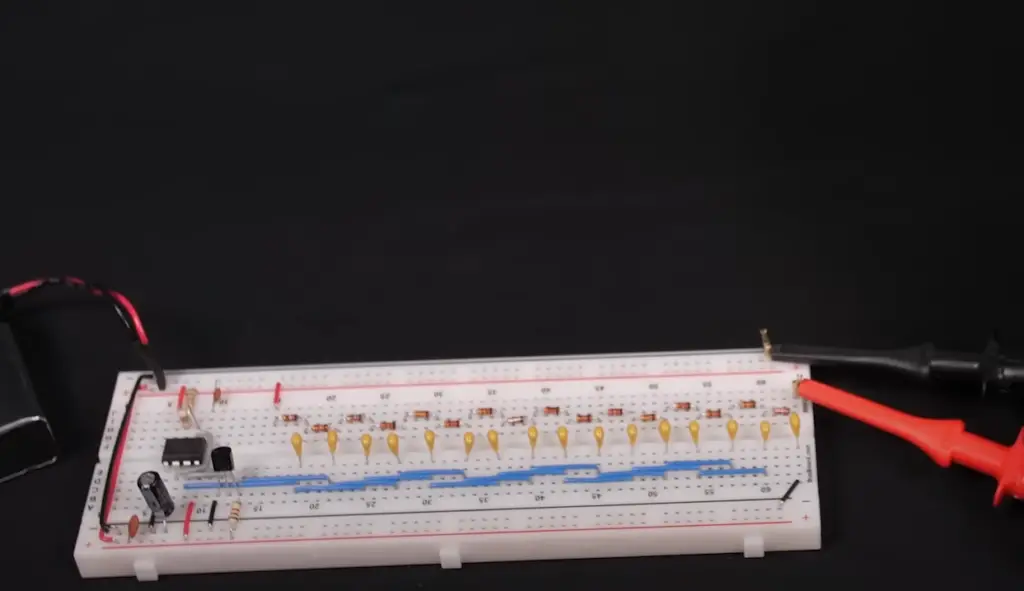• High accuracy and precision – The use of two or more capacitors enables greater accuracy and precision than other voltage dividers. For example, when compared to resistor-based dividers, the capacitive voltage divider produces results which are usually within 1 percent error range.
• Low power consumption – Capacitive voltage dividers require very little power and can be used in applications where power consumption must be kept to a minimum.
• Low resistance – Capacitors have less electrical resistance than resistors, which results in less energy being dissipated from the circuit as heat.

• High cost – The components of a capacitive voltage divider are relatively expensive when compared to other types of dividers.
• Sensitivity to temperature changes – The output of the divider is sensitive to changes in ambient temperature, which can cause inaccurate results if not properly accounted for.
• Complexity – A capacitive voltage divider requires careful design and calculation in order to obtain optimal results. This complexity can make it difficult to use in certain applications.

Overall, a capacitive voltage divider can provide greater accuracy and precision than other types of dividers at the cost of being more expensive and complex. It is important to carefully consider the advantages and disadvantages when deciding if this type of circuit is right for your application. 

## Applications

The capacitive voltage divider is an essential and versatile circuit that has many applications. It can be used in power supplies, signal processing circuits, transducers, amplifiers, oscillators and audio circuits. It is also commonly used to measure alternating current (AC) signals in sensitive electronic equipment such as computers, medical instruments and radio receivers. Its ability to provide a wide range of voltage gain makes it particularly useful for controlling the level of AC inputs in these devices. Additionally, the capacitive voltage divider can be used to measure the phase difference between two or more AC signals and to provide adjustable amplitude control to audio systems. The versatility of this circuit makes it a valuable tool in the world of electronics engineering.

In addition to its many uses in electronic engineering, the capacitive voltage divider can also be used in industrial and home applications. In order to monitor the power consumption of a device or appliance, for instance, a capacitive voltage divider may be utilized to measure the AC voltage level at different points within the circuit. This type of measurement is important in order to regulate power flow and prevent overloads. Additionally, it may also be used as part of an energy management system that limits electrical loads when necessary.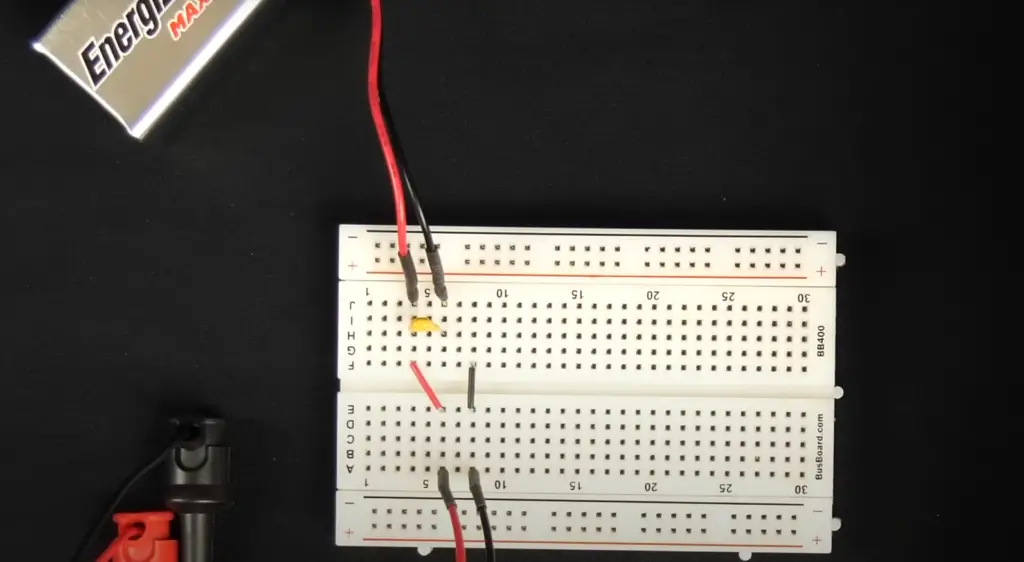## What is a Voltage Divider Rule?

The Voltage Divider Rule is a fundamental law of electrical circuits that states the voltage (V) across a resistor in series with two other resistors is equal to the ratio of their resistance multiplied by the total voltage. In other words, V₁ = (R₁/(R₁+R₂))*Vtotal.

This can be used to create a simple circuit known as a voltage divider, or capacitive voltage divider.

A capacitive voltage divider consists of two series-connected resistors connected to a capacitor. When an alternating current is applied to this circuit, the capacitor’s impedance and phase angle will affect its output voltage. The output voltage will be lower than the input voltage, and the capacitive voltage divider can be used to adjust this ratio. It is especially useful when a certain output voltage needs to be maintained regardless of changes in the input voltage.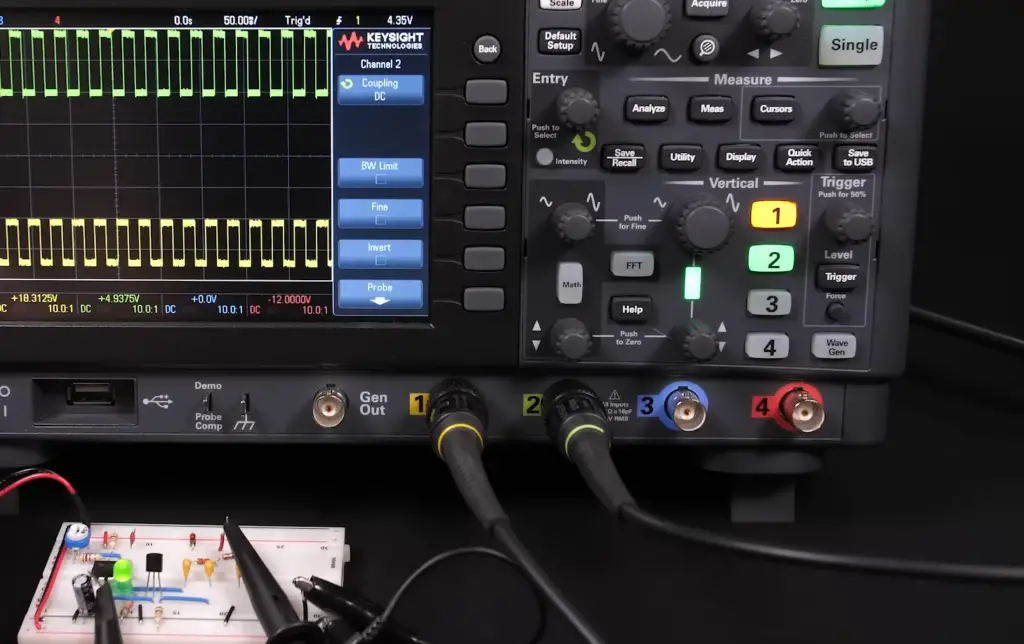## FAQ

### What is the meaning of capacitive voltage divider?

A capacitive voltage divider is a circuit composed of two or more series connected capacitors.

The output of the circuit is a fraction of the input that is determined by the ratio between the capacitances of the two capacitors. This allows for voltage division across its components in order to measure, control and provide an accurate reference for circuits.

### What are some applications of a capacitive voltage divider?

Capacitive voltage dividers can be used in various applications such as sensor conditioning, filter design, signal isolation and power supply decoupling. They are also commonly used in high-precision current measurement systems and frequency generators. Additionally, they can be used to generate precise split voltages with low source impedance which is useful for analog-to-digital conversion and A/D calibration.

### What are the advantages of a capacitive voltage divider?

The main advantage of a capacitive voltage divider is its precision, accuracy and stability due to the low temperature coefficient associated with the capacitor elements. It also has low power consumption and can be used in wide range of operating temperatures. Additionally, this type of voltage divider has high input impedance which makes it suitable for measuring small signals in noisy circuits. Furthermore, they have fast settling times compared to resistive dividers making them ideal for applications requiring quick response. Finally, they have good noise immunity which allows them to function properly even in electrically noisy environments.

### What is the rule of capacitive voltage divider?

The rule of capacitive voltage divider states that the ratio of a capacitor’s voltage across its two terminals to the total applied voltage is equal to the ratio of the capacitance of that capacitor compared to the sum of all the capacitances in series. In other words, if there are two capacitors (C1 and C2) connected in series with an applied voltage V, then V1/V = C1/(C1 + C2). This rule can be used for many types of circuits featuring capacitors in parallel or series configurations. The rule allows for more accurate predictions about circuit behavior since it takes into account not only resistive characteristics but also those related to electric fields. Additionally, this law helps engineers design better circuits by providing them with a precise way to calculate potential voltage drops across different components.

### What is the difference between current divider and voltage divider?

The main difference between a current divider and a voltage divider is the factor that affects their operation. A current divider is affected by the electrical resistance (resistance to electric current flow) of each component in the circuit, while a voltage divider is affected by the capacitance (ability to store electric charge) of its components. A capacitive voltage divider works on the principle that when two capacitors are connected in series across a dc source, one capacitor will draw more current than the other. The ratio of these currents depends on the capacitances of each capacitor as well as any other impendences present in the system. This allows for an adjustable voltage division between them, thus creating what is known as a capacitive voltage divider. This circuit is useful for many applications ranging from AC/DC converters to voltage regulators.

### Is a parallel circuit a voltage divider?

No, a parallel circuit is not a voltage divider. A parallel circuit does not have the same type of current-splitting behavior as a voltage divider. Instead, a parallel circuit will distribute power evenly among its branches and components, meaning that each branch and component in the circuit will experience the same amount of voltage from the source. However, it is possible to use capacitors in a parallel circuit to create something similar to what would be found in a capacitive voltage divider. This can be done by connecting two identical capacitors across the source with opposite polarity.

### Why do capacitors in parallel have equally divided voltage?

Capacitors in parallel will divide the voltage because of their different capacitances. Since a capacitor’s capacitance is determined by its size and shape, two identical capacitors with different shapes or sizes will have different amounts of capacitance. This difference in capacitance causes each capacitor to draw a different amount of current from the source, resulting in an unequal distribution of voltage between them. This can be used to create a voltage divider circuit when these two differently sized capacitors are connected together in parallel across a dc source. The ratio of voltage at each node depends on the individual capacitor’s capacitance and any other impedances present in that portion of the circuit.

## Conclusion

A capacitive voltage divider is a passive electronic component used to convert an input voltage into two separate output voltages, one of which is higher than the other. It does this by using capacitors as resistive elements in order to divide the input voltage between them. This type of circuit can be used for various applications such as signal conditioning, power supply regulation, and noise reduction. Its simple construction and low cost make it an attractive choice for many electronic projects. Additionally, since it uses capacitance instead of resistance, its performance is not affected by changes in temperature or humidity.

With proper design and application knowledge, a capacitive voltage divider can be a very useful tool for engineers and hobbyists alike.